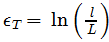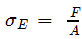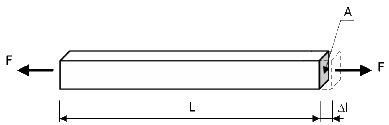# Defining Stress-Strain Curves

Stress-strain curves are available with the following material models: Nonlinear Elastic, Plasticity - von Mises, and Plasticity - Tresca.

To define a stress-strain curve:

1. In the Material dialog box, right-click Custom Materials and select New Category. If needed, rename the New Category folder.
2. Right-click the newly defined category and select New Material.
3. On the Properties tab, do the following:
1. Set Model Type to either Nonlinear Elastic, Plasticity - von Mises, or Plasticity - Tresca.
2. Select the desired Units.
3. Click Create Stress-Strain Curve.
The Tables & Curves tab is active and Stress-Strain Curve is selected in Type.
4. In Table data, do the following:
1. Select the desired stress in Units.
2. Enter the strain and stress data pairs in the table. For large strain formulation, enter the logarithmic strain data.
3. To open a new row, double-click any cell in the Points column.
4. Click File to read data from a *.dat text file with two columns of data.
5. Click View to view the curve.
5. To save the material to a material library, click Save.
6. Click Apply.
7. Click Close.
• During the solution, when values for the stress-strain data exceed the last datapoint of the curve, the software extrapolates linearly the last couple of data points on the stress-strain curve you enter.
• For Hyperelastic Ogden and Hyperelastic Mooney Rivlin material models, you can define stretch ratio (deformed length/undeformed length) versus nominal stress (force/initial area) data pairs based on experimental data from specimen tests: simple tension, planar tension or pure shear, and biaxial tension.

## Input for Stress/Strain Curves

Depending on the settings, nonlinear analysis may require the input of stress/strain curves. When that is the case, the curve should be entered using the correct definitions for stress and strain.

The table below summarizes the types of stress and strain to be used as input for the stress/strain curve, depending on the analysis option and the type of material model used.

Analysis Options
Material Model Small Strain, Small Displacement Small Strain, Large Displacement Large Strain, Large Displacement
Non Linear Elastic True Stress, Engineering Strain True Stress, Engineering Strain N/A
Elasto - Plastic von Mises Plasticity, Tresca Plasticity, Drucker Prager True Stress, Engineering Strain True Stress, Engineering Strain True Stress, Logarithmic Strain
Hyper Elastic: Mooney-Rivlin, Ogden Blatz Ko Engineering Stress, Stretch ratio Engineering Stress, Stretch ratio Engineering Stress, Stretch ratio
Super Elastic True Stress, Logarithmic Strain True Stress, Logarithmic Strain True Stress, Logarithmic Strain
Viscoelastic True Stress, Engineering Strain True Stress, Engineering Strain N/A

After the analysis completes, the stress output is the Cauchy stress, which is the true stress in the deformed geometry.

The strain output depends on the material model and the choice of small or large strain formulation.

For non-linear elastic models: von Mises Plasticity, Tresca Plasticity, Drucker Prager, Super Elastic, and Viscoelastic the small strain option produces engineering strains; the large strain option produces logarithmic strains.

 True Stress and Strain If the deformation of a bar in tension becomes significant, its cross-sectional area will change. The traditional engineering definitions for stress and strain are no longer accurate and new measures, namely true stress and true strain, are introduced. Alternative names for these quantities are Cauchy stress, logarithmic strain, and natural strain.The true stress is, where a is the final deformed cross-sectional area. The true strain is, where l is the final length and L is the initial undeformed length of the bar.Engineering Stress and Strain The engineering stress (or nominal stress), where A is the initial undeformed cross-sectional area. The engineering strain (or nominal strain) iswhere Δl is the final bar deformation.• Engineering strain is a small strain measure which is invalid once the strain in your model is no longer "small" (approximately larger than 5%). Logarithmic strain, which is a nonlinear strain measure that is dependent upon the final length of the model, is used for large strain simulations.
• For viscoelastic material model, the definition of stress versus strain is replaced by relaxation function versus time.
• Extrapolation of stress/strain curve after the curve's last data points: for plasticity or nonlinear elastic material definition the last couple of data points are extrapolated linearly to calculate pairs of data points outside the defined stress/strain curve.
• When you define a stress-strain curve, the first point on the curve should be the yield point of the material. Material properties like elastic modulus, Yield strength, etc will be taken from the stress-strain curve when it is available and not from the material properties table in the Material dialog box. Only the Poisson's ratio (NUXY) will be taken from the table.